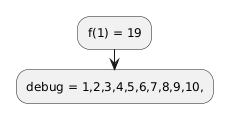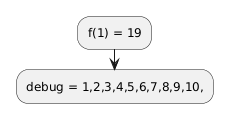# some recursive functions don't work as expected

Playing around with recursive preprocessor functions uncovers a strange bug.  I can't quite work out what it is doing, but here's my best attempt at demonstrating:

Take a function f(x) = f(x + 1) + 1 & f(10)=10.  I would expect f(1) = f(2)+1 = f(3)+2 = f(4)+3 = ... = f(10)+9 = 19

But coding it in Plantuml gives the answer 11...  So I stuck in some additional debug info:

```@startuml
!function \$f(\$n)
!\$debug=\$debug + \$n +","
!if (\$n >= 10)
!return \$n
!else
!return \$f(\$n + 1) + 1
!endif
!end function

!\$debug = ""
:f(1) = \$f(1);
:debug = \$debug;
@enduml```This suggests that the function is getting called only 6 times (instead of 10 times) and each call is n+2 instead of n+1.

My best guess is that

`!return \$f(\$n + 1) + 1`

is somehow getting processed as

`!return \$f(\$n + 1 + 1)`

I can 'fix' it using %intval(), as follows;

```@startuml
!function \$f(\$n)
!\$debug=\$debug + \$n +","
!if (\$n >= 10)
!return \$n
!else
!return %intval(\$f(\$n + 1)) + 1
!endif
!end function

!\$debug = ""
:f(1) = \$f(1);
:debug = \$debug;
@enduml
```Your name to display (optional): Email me at this address if my answer is selected or commented on: Privacy: Your email address will only be used for sending these notifications. Anti-spam verification:[Antispam2 Feature: please please wait 1 or 2 minutes (this message will disappear) before pressing the button otherwise it will fail]`(--------)` To avoid this verification in future, please log in or register.